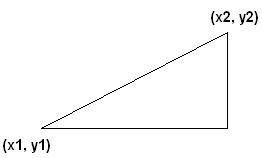# 3. Maths Lines

I am going to start this tutorial now, but I probably will not have time to finish it. But here goes…

The equation of a line can be shown in 2 different forms. There is the General Equation and the Slope-Intercept form. They are:

General Equation: aX + bY = C
Slope-Intercept form: Y = mX + C

I find the slope intercept form best, as it tells you straight out where the formula crosses the y axis at X and the slope of the line is M.

Let us reuse this diagram:Now first we will look at the slope:
The formula for a slope between 2 points is:
M = y2-y1
——
x2-x1

Simple enough. Now to find C, we use the equation Y = mX + C:
(y2) = M * (x2) + C

So we are substituting in one of the set of points, also adding in M which we solved before.

so you end up with:
(y2)
—– – M = C
(x2)

Now that we have both C and M we can determine that the formula is:
y = mX + C by adding in the numbers for C and M.

Now that we have our formula in the slope-intercept form, what about converting that to the General form?

Y = mX + C

Y
— – X = C
M

I will add more onto this later, as it is a tad longer than the others. Stay tuned….

If you have any questions, please email me at swiftless@gmail.com

• March 25, 2010
• 3